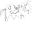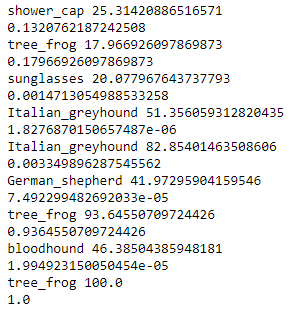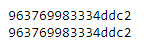pico18 Dog or Frog

Misc - 900

### Description

This is a classic machine learning problem.

We came across this article and copy much of the template code from there. I wrote my code in a Jupyter notebook.

The only problem that I encountered was that of the learning rate. The example’s learning rate was way too small, and resulted in no progress. In order to solve, I set the learning rate to 1e7 and decayed it by 0.99 per iteration. Although these hyperparameters can probably be tuned better, these result in a solve in about 15 seconds with 100% accuracy.

### Sample training### Error

This method is sufficient to minimize error, as shown below.

def hash_hamming_distance(h1, h2):
s1 = str(h1)
s2 = str(h2)

print(s1)
print(s2)

return sum(map(lambda x: 0 if x == x else 1, zip(s1, s2)))

def is_similar_img(path1, path2):
image1 = Image.open(path1)
image2 = Image.open(path2)

dist = hash_hamming_distance(phash(image1), phash(image2))
return dist <= 2

assert is_similar_img("./img/trixi.png", "./hacked-image.png")